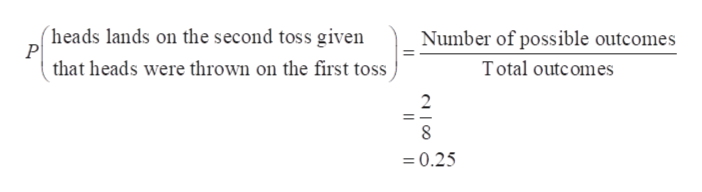# A coin is tossed 3 times. What is the probability that the coin will land heads:A) atleast twice?B) on the second toss given that heads were thrown on the first toss?C) on third toss given that tails were thrown on the first toss?

Question

A coin is tossed 3 times. What is the probability that the coin will land heads:

A) atleast twice?

B) on the second toss given that heads were thrown on the first toss?

C) on third toss given that tails were thrown on the first toss?

check_circle

Step 1

Consider H represent the outcome of heads and T represents the outcome of tails. The total number of outcomes after tossing a coin 3 times can be obtained as:

HHH, HHT, HTH, HTT, THH, THT, TTH, TTT

So, the total outcomes are 8.

a)  The total choices that heads will land at least twice are HHH, HHT, HTH, THH that is 4.

The required probability that heads will land at least twice can be obtained as:

Step 2

b) The possible outcomes that heads lands on the second toss given that heads were thrown on the first toss are HHH, HHT.

The requ...help_outlineImage Transcriptionclose(heads lands on the second toss given P Number of possible outcomes that heads were thrown on the first toss Total outcomes 2 8 0.25 fullscreen

### Want to see the full answer?

See Solution

#### Want to see this answer and more?

Solutions are written by subject experts who are available 24/7. Questions are typically answered within 1 hour.*

See Solution
*Response times may vary by subject and question.
Tagged in

### Basic Probability